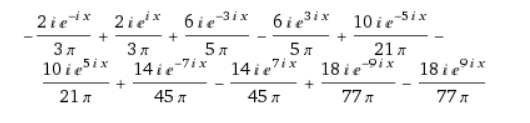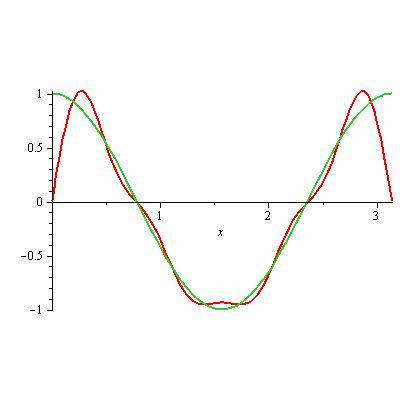# Fourier sine series of cos 2x

Morbidly_Green
Homework Statement:
Find the Fourier sine series of cos 2x on [0, π]
Relevant Equations:
$$b_n = \dfrac{2}{l} \int^\pi _0 f(x) sin(\dfrac{n\pi x}{l})dx$$
I am attempting to find the sine representation of cos 2x by letting

$$f(x) = \cos2x, x>0$$ and $$-\cos2x, x<0$$

Which is an odd function. Hence using $$b_n = \dfrac{2}{l} \int^\pi _0 f(x) \sin(\dfrac{n\pi x}{l})dx$$ I obtain $$b_n = \dfrac{2n}{\pi} \left( \dfrac{(-1)^n - 1}{4-n^2} \right)$$

which when I plot the sine series

$$\sum^\infty _{n=3} \dfrac{2n}{\pi} \left( \dfrac{(-1)^n - 1}{4-n^2} \right) \sin(n x)$$

I don't get the fn of cos 2x on [0, π]. I don't understand where I went wrong any help would be great.

Homework Helper
Don't follow: you make an odd continuation of ##f(x)## but don't actually use it: your bounds are 0 and ##\pi##

Morbidly_Green
Don't follow: you make an odd continuation of ##f(x)## but don't actually use it: your bounds are 0 and ##\pi##
Yes, this is true, but I used the formula for $$b_n$$ that assumes I do indeed have an odd function

Homework Helper
So basically you have only forced ##\ f(0) = f(\pi)=0\ ## as is necessary with a sine series.

(and integrating from ##\pi## to ##2\pi## gives the same as from 0 to ##\pi## anyway)

I tried a cheat and got (for n up to 9):so it looks your result is fine. Why do you think otherwise ?

Morbidly_Green
When I plotted the Fourier series on top of the function cos2x they did not match

So basically you have only forced ##\ f(0) = f(\pi)=0\ ## as is necessary with a sine series.

(and integrating from ##\pi## to ##2\pi## gives the same as from 0 to ##\pi## anyway)

I tried a cheat and got (for n up to 9):

View attachment 241922
so it looks your result is fine. Why do you think otherwise ?

Homework Helper
When I plotted the Fourier series on top of the function cos2x they did not match
Like 'not at all', 'not very well', 'not perfectly' ?

Did you check the link ? it has a plot too ...

To get a good match you need an awful lot of terms: the function has to jump from 0 to 1 in a step of 'size zero' at ##x=0##

Homework Helper
Gold Member
Edit[added]: I didn't notice your sum started at n=3. Anyway here's a plot to compare with.

@Morbidly_Green: Your problem is your formula for ##b_n## when ##n=2##. You need to calculate ##b_2## separately, and when you do, you will find than ##b_2=0##. Once you fix that, your plot should look better. Here's what I get for the first 10 terms:Morbidly_Green
Edit[added]: I didn't notice your sum started at n=3. Anyway here's a plot to compare with.

@Morbidly_Green: Your problem is your formula for ##b_n## when ##n=2##. You need to calculate ##b_2## separately, and when you do, you will find than ##b_2=0##. Once you fix that, your plot should look better. Here's what I get for the first 10 terms:
View attachment 241924
Ah yes! Thank you, I completely forgot that you can't just ignore the first terms !

Homework Helper
In particular ##b_1## ! Why did you start at ##n=3## ?

Morbidly_Green
In particular ##b_1## ! Why did you start at ##n=3## ?
Since n=2 would yield a division by zero I started from the first calculable term, n=3, and as I said I forgot how important the terms before are

Homework Helper
OK, thanks. All in all: well done !

Homework Helper
Dearly Missed
I am attempting to find the sine representation of cos 2x by letting

$$f(x) = \cos2x, x>0$$ and $$-\cos2x, x<0$$

Which is an odd function. Hence using $$b_n = \dfrac{2}{l} \int^\pi _0 f(x) \sin(\dfrac{n\pi x}{l})dx$$ I obtain $$b_n = \dfrac{2n}{\pi} \left( \dfrac{(-1)^n - 1}{4-n^2} \right)$$

which when I plot the sine series

$$\sum^\infty _{n=3} \dfrac{2n}{\pi} \left( \dfrac{(-1)^n - 1}{4-n^2} \right) \sin(n x)$$

I don't get the fn of cos 2x on [0, π]. I don't understand where I went wrong any help would be great.

You need to keep ##a_1, a_3, a_4, \ldots##. You could also include ##a_2##, but you cannot use the general form for ##a_n##: you need to put ##a_2=0## manually.